# Grove Beginner Kit for Arduino Projects

## Project 1: Intrusion Alarm​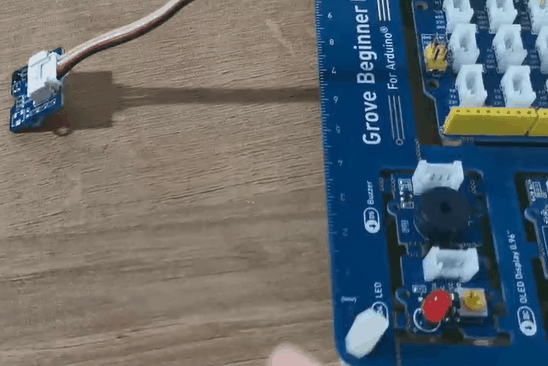Overview

This wiki introduces how to make a intrusion alarm.

Feature

• The PIR motion sensor can detect people if in the area, then alarm triggered.

Component required

Hardware Connection

Please follow the same color line to connect each sensor on the board, put the PIR motion sensor grove cable to the D2.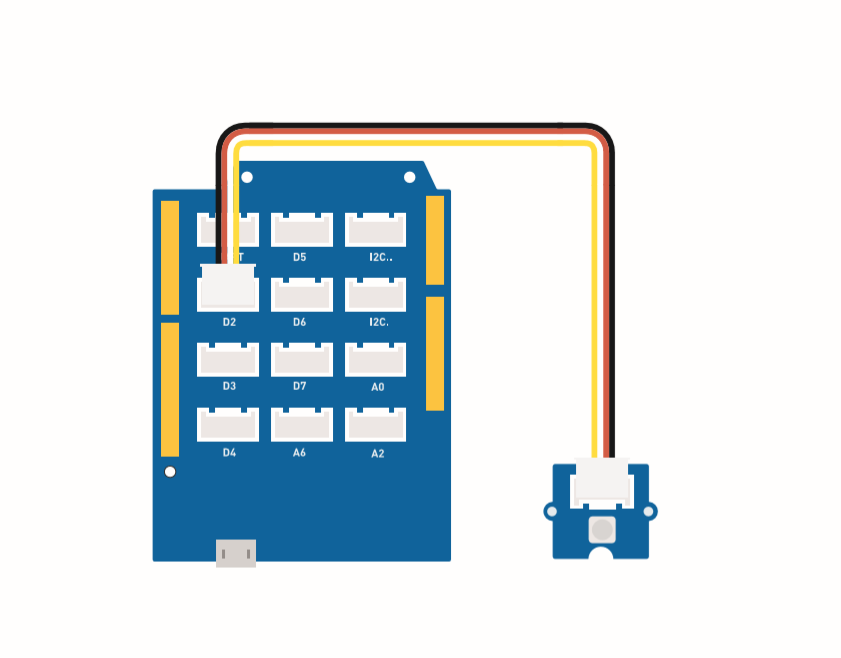Assembly instructions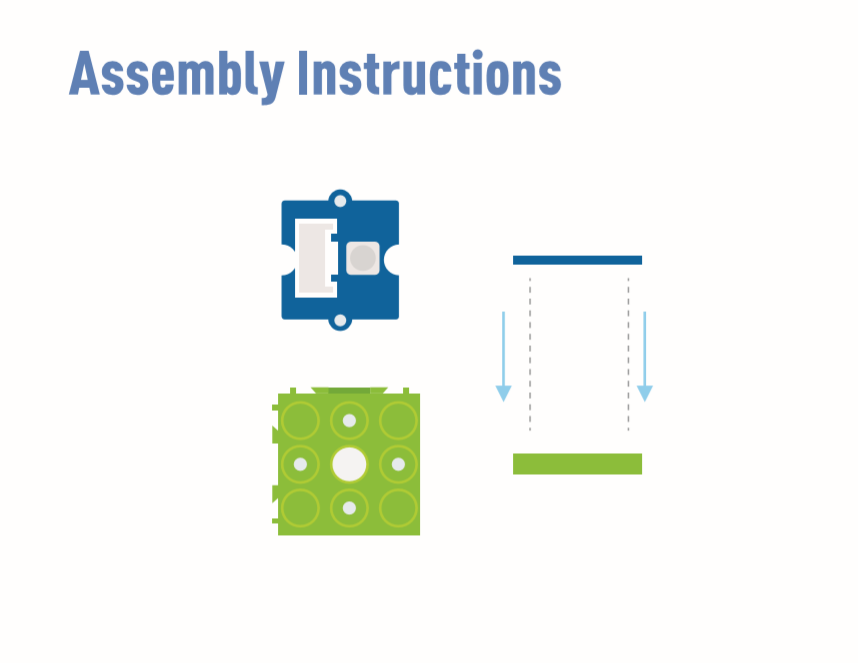note
``The buzzer (D5) and LED (D4) are embedded in the board.``

Arduino Instructions

Step 2. Follow the connection picture connect all the sensor on the board.

Step 3. Place the Mini PIR motion sensor in the location of the detection.

Step 4. Copy the code stick on the Aruino IDE then upload it.

Code

``#define PIR_MOTION_SENSOR 2//Use pin 2 to receive the signal from the moduleint BuzzerPin = 5;     // set D5 as buzzerint LED_RAD = 4;       // set D4 as LEDvoid setup() {  Serial.begin(9600);  pinMode(PIR_MOTION_SENSOR, INPUT);  pinMode(BuzzerPin, OUTPUT);  pinMode(LED_RAD, OUTPUT);}void loop() {  if (digitalRead(PIR_MOTION_SENSOR)) {    analogWrite(BuzzerPin, 100);    digitalWrite(LED_RAD, HIGH);    delay(3000);    analogWrite(BuzzerPin, 0);    digitalWrite(LED_RAD, LOW);    delay(4000);  }}``

## project 2: Oscillating fan​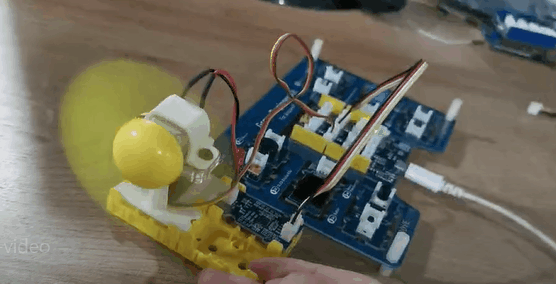Overview

This wiki introduce how to make a Mini fan to plase on your room keep cool.

Feature

• Automatic swing fan

Component required

Hardware Connection

Please connect the fan grove cable to D7, Servo grove cable to D3.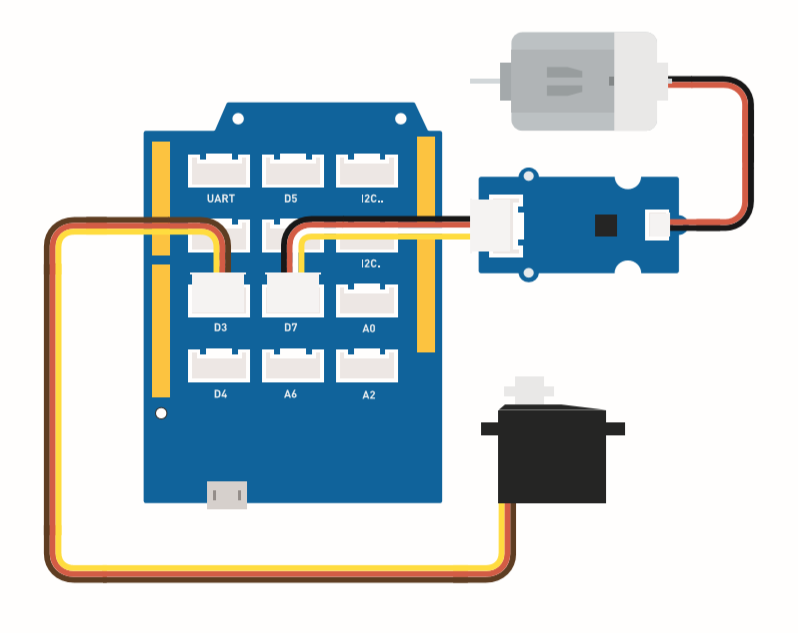Assembly instruction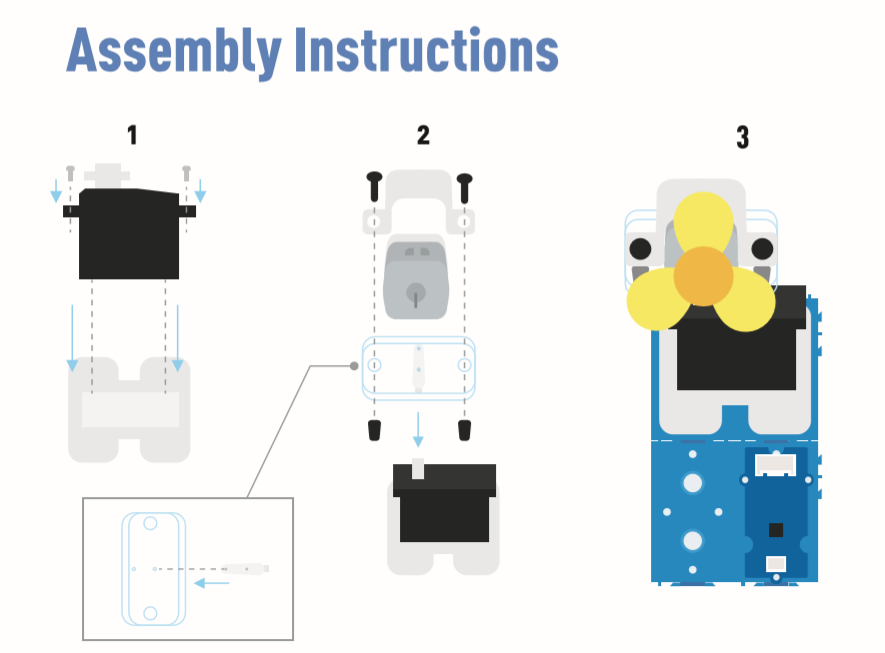Arduino Instructions

Step 2. Copy the Code and stick on the Arduino IDE

Step 3. Select the current port: Tools -> Port -> COM (number)

note
``Please set the fan in the safety position.``

Code

``#include <Servo.h>Servo myservo;  // create servo object to control a servoint pos = 0;    // variable to store the servo positionint fanPin = 7;  // set D6 as control switch int fanState = LOW;void setup() {    Serial.begin(9600);    myservo.attach(3);  // attaches the servo on pin 2 to the servo object    pinMode(fanPin, OUTPUT);} void loop() { fanState = HIGH; digitalWrite(fanPin, fanState);   for (pos = 0; pos <= 100; pos += 1) { // goes from 0 degrees to 100 degrees    // in steps of 1 degree    myservo.write(pos);              // tell servo to go to position in variable 'pos'    delay(40);                       // waits 15ms for the servo to reach the position  }  for (pos = 100; pos >= 0; pos -= 1) { // goes from 100 degrees to 0 degrees    myservo.write(pos);              // tell servo to go to position in variable 'pos'    delay(40);                       // waits 15ms for the servo to reach the position  }}``

## Project 3: Remote Control Oscillating Fan​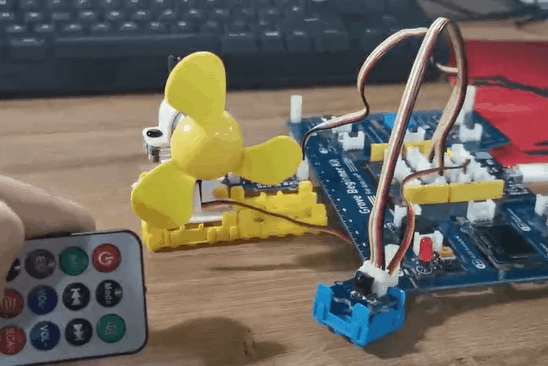Overview

This wiki introduces how to make a remote control oscillating fan.

Feature

• The fan power controlled by the controller.

• The fan swing way is able to use remote control.

Component required

Hardware Connection

Please follow the same color line to connect each sensor on the board. Please connect the fan grove cable to D7, servo grove cable to D3, IR grove cable to D2.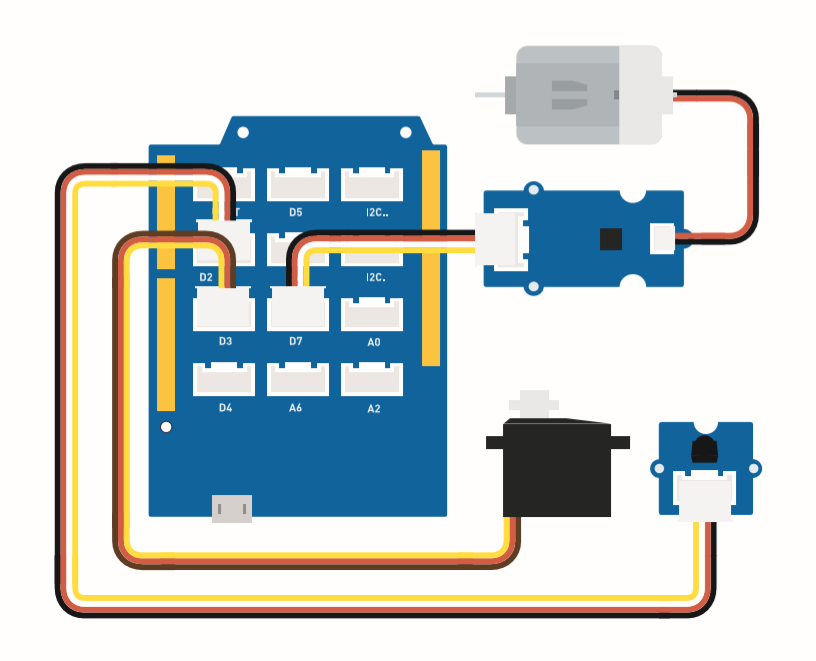This is controller botton function.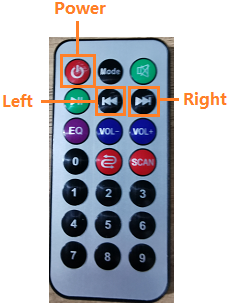Assembly instruction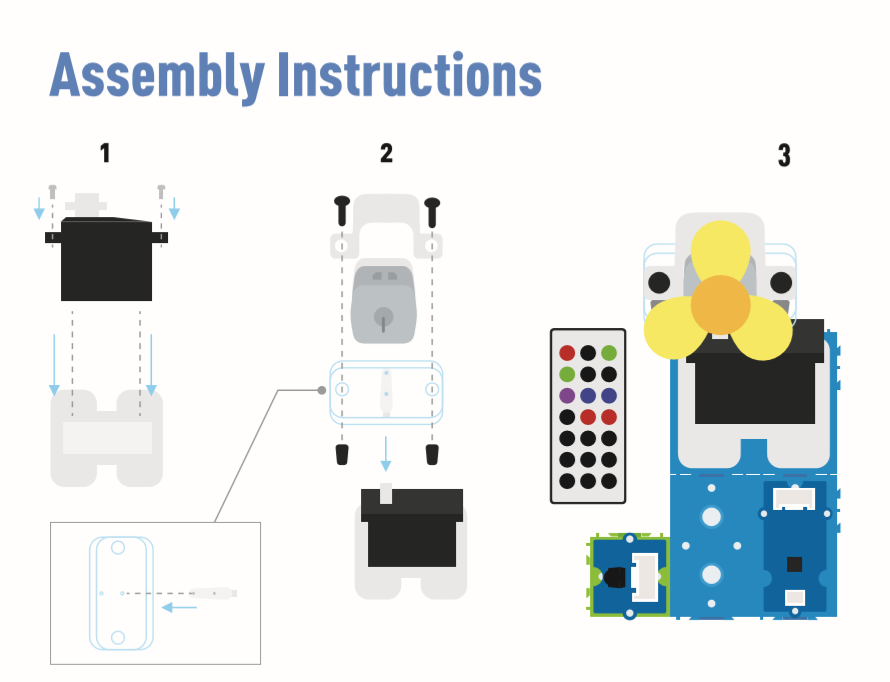Arduino Instructions

Step 1. Follow the connection picture connect all the sensor on the board.

Step 3. Navigate to Sketch -> Include Library -> Manage Libraries, search IRremote then install it.

Step 4. Copy the code stick on the Aruino IDE then upload it.

Step 5. Place the Fan in the safety position, try to press the button make sure it can work safely.

note
``Refer How to [**install library**](https://wiki.seeedstudio.com/How_to_install_Arduino_Library) to install library for Arduino.``

Code

``#include <IRremote.h>#include <Servo.h>Servo myservo;  // create servo object to control a servoint RECV_PIN = 2; // set pin 2 as IR controlIRrecv irrecv(RECV_PIN);decode_results results;int pos = 90;    // variable to store the servo positionint fanPin = 7;  // set D6 as control switchint fanState = LOW;int IO = 0;void setup(){  Serial.begin(9600);  Serial.println("Enabling IRin");  // remind enabling IR  irrecv.enableIRIn(); // Start the receiver  Serial.println("Enabled IRin");  myservo.attach(3);  // attaches the servo on pin 2 to the servo object  pinMode(fanPin, OUTPUT);}//  power_encode 2155829415     left  2155870215  right  2155821255void loop() {  if (irrecv.decode(&results)) { //checking IR signal    if (results.value == 2155829415) {    // Power off/on      IO++;      if (IO % 2 == 0) {        fanState = HIGH;        digitalWrite(fanPin, fanState);        delay(100);      }      else {        fanState = LOW;        digitalWrite(fanPin, fanState);        delay(100);      }    }    if (results.value == 2155821255 ) {    // fan swing to left      for (pos; pos <= 89; pos += 1) { // goes from 0 degrees to 90 degrees        // in steps of 1 degree        myservo.write(pos);              // tell servo to go to position in variable 'pos'        delay(40);                       // waits 15ms for the servo to reach the position        if (irrecv.decode(&results)) {          irrecv.resume();          if (results.value == 2155870215)            break;        }      }    }    if (results.value == 2155870215 ) {    // fan swing to right      for (pos; pos >= 1; pos -= 1) { // goes from 90 degrees to 0 degrees        myservo.write(pos);              // tell servo to go to position in variable 'pos'        delay(40);                       // waits 15ms for the servo to reach the position        if (irrecv.decode(&results)) {          irrecv.resume();          if (results.value == 2155821255)            break;        }      }    }    Serial.println(pos);    Serial.println(results.value, HEX);    Serial.println(results.value);    irrecv.resume();                    //recive next intrustion  }  delay(100);}``

## Project 4: Smart Humidifier​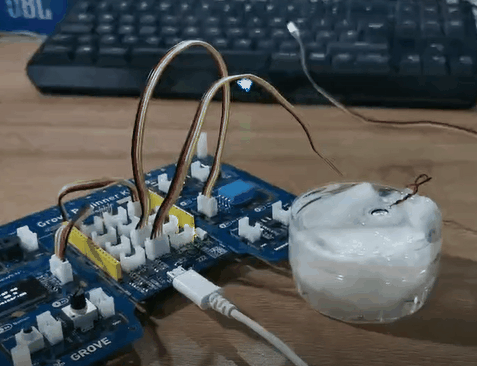Overview

This wiki introduces how to make water atomization to keep indoor humidity normal.

Feature

• Automatically use the water atomization when the humidity is low.

• Display the temperature and humidity in real-time.

Component required

Hardware Connection

Please follow the same color line to connect each sensor on the board. Connect the Grove water atomization cable to D2.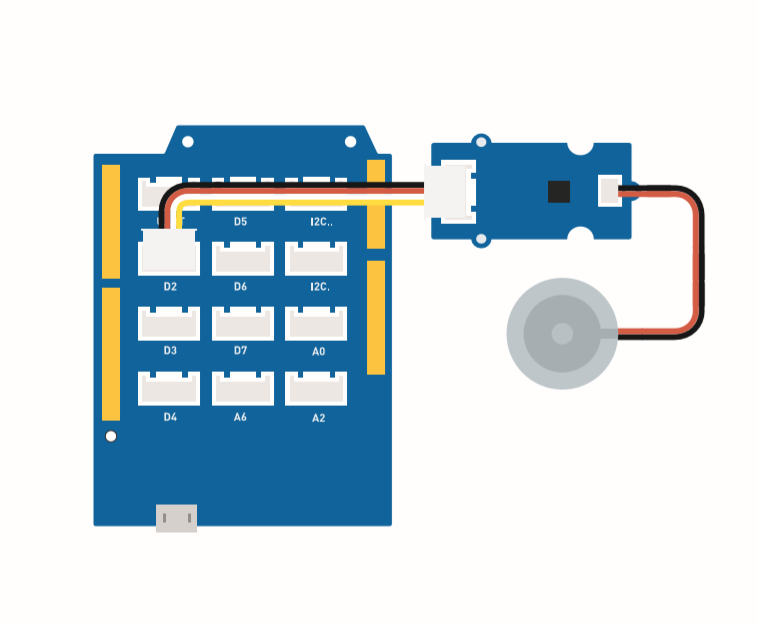Assembly instructions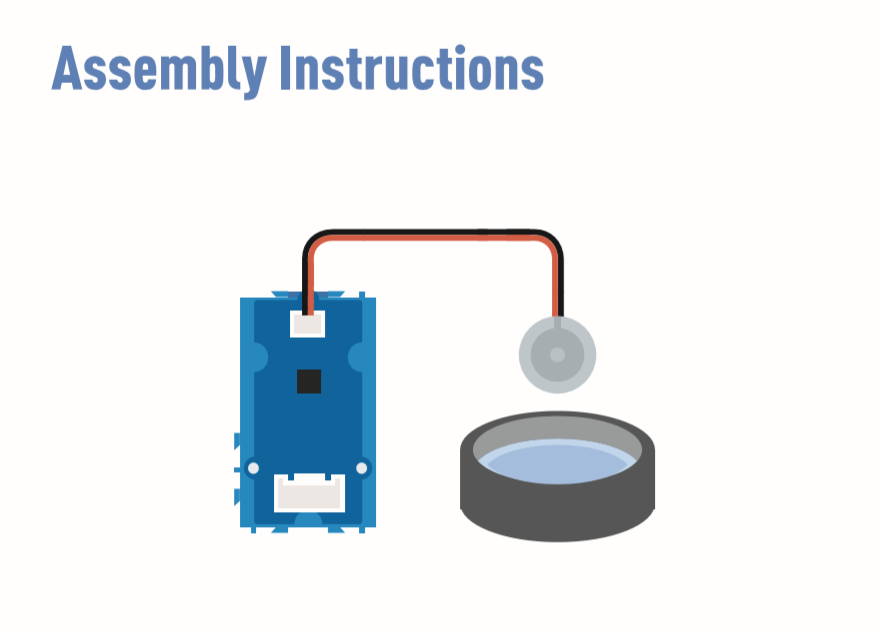Arduino Instructions

Step 1. Follow the connection picture connect all the sensor on the board.

Step 3 Navigate to Sketch -> Include Library -> Manage Libraries, search U8g2 then install it.

Step 5. Copy the code stick on the Aruino IDE then upload it.

Step 6. Prepare a contain with full water then put the water atomization on the water.

note
``Refer How to [**install library**](https://wiki.seeedstudio.com/How_to_install_Arduino_Library) to install library for Arduino.``
note
``Prepare some tissue put on the water, let the water atomization keep afloat. The function of tissue is lead water to the transducer and keep upper side of transducer above water.``

Code

``#include <Arduino.h>#include <U8x8lib.h>#include "DHT.h"#define DHTTYPE DHT11   // DHT 11#define DHTPIN 3     // what pin we're connected toDHT dht(DHTPIN, DHTTYPE);#include <Wire.h>U8X8_SSD1306_128X64_NONAME_HW_I2C u8x8(/* reset=*/ U8X8_PIN_NONE);void setup(void) {  Serial.begin(115200);  u8x8.begin();  u8x8.setFlipMode(1);  Wire.begin();  dht.begin();  pinMode(2,INPUT);} void loop(void) {  float temp_hum_val = {0};  int b;  int c;  if (!dht.readTempAndHumidity(temp_hum_val)) {     b = temp_hum_val;     c = temp_hum_val;  }  else{    Serial.println("Failed to get temprature and humidity value.");    }  u8x8.setFont(u8x8_font_chroma48medium8_r);   // choose a suitable font  u8x8.setCursor(0, 0);  u8x8.print("Temp: ");    u8x8.setCursor(5, 0);  u8x8.print(c);    u8x8.setCursor(8, 0);  u8x8.print("*C");    u8x8.setCursor(0, 10);  u8x8.print("Hum: ");    u8x8.setCursor(5, 10);  u8x8.print(b);    u8x8.setCursor(8, 10);  u8x8.print("%");    u8x8.setCursor(0, 20);  u8x8.print("atomizer: ");    if(b > 70){  u8x8.setCursor(9, 20);  u8x8.print("OFF");    digitalWrite(2, LOW);    // atomization stopped  }  if(b <= 70) {  u8x8.setCursor(9, 20);  u8x8.print("ON ");   digitalWrite(2, HIGH);   // atomize     }  delay(1000);  }``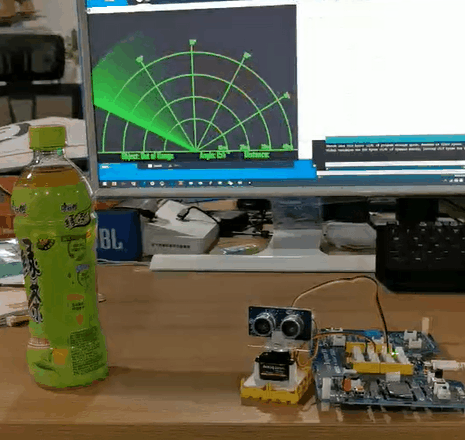Overview

This wiki introduce how to make a Ultrasonic Radar to detect the object and distance.

Feature

• Detect the object distance

• Scan if exist the object in around

Component required

Hardware Connection

Please follow the picture, connect the ultrasonic sensor Grove cable to the D2, connect the servo to the D7.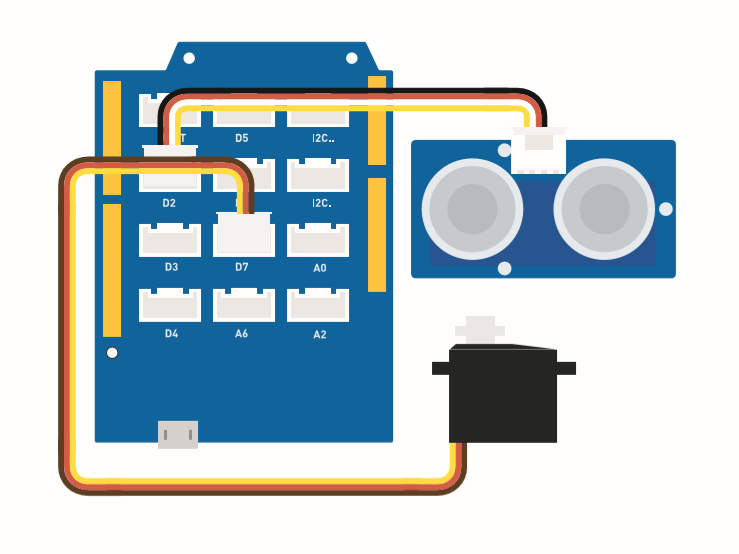Assembly instuction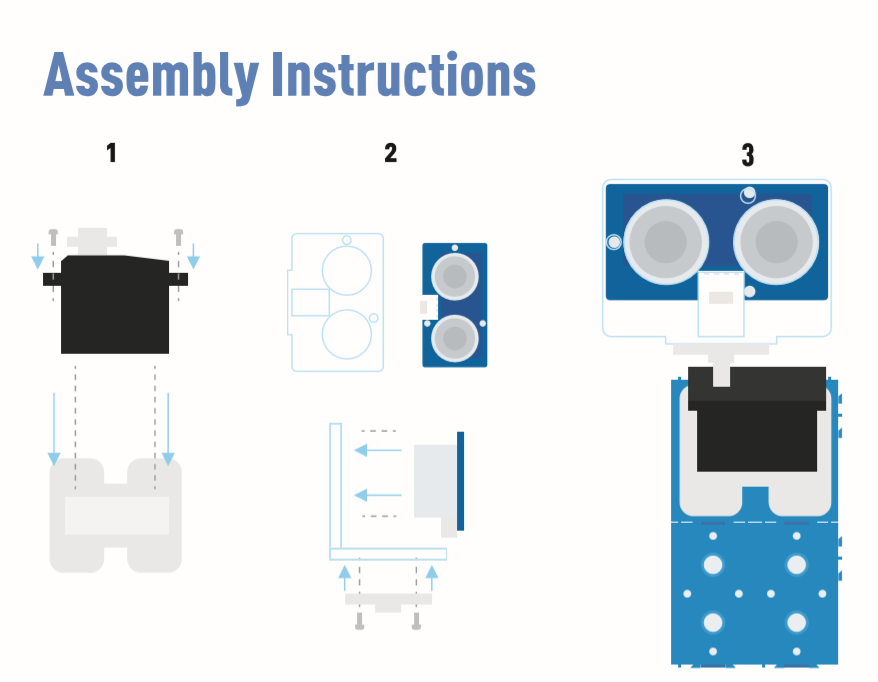Arduino Instructions

Step 1. Follow the Connection to plug the cable on the port.

Step 5. Copy the Radar code and stick on the Arduino IDE then upload it.

Step 7. Copy the Radar-Processing Code and stick on the Processing.

Step 8. After the Servo start swing, click play on the Processing software.

note
``If you do not konw how to intall the library, Click [**here**](https://wiki.seeedstudio.com/Grove-Ultrasonic_Ranger/#software).``

``#include <Servo.h>#include "Ultrasonic.h"int distance;Servo myServo;Ultrasonic ultrasonic(2);void setup() {    Serial.begin(9600);    myServo.attach(7);}void loop() {    for(int pos = 15; pos <= 165; pos += 1){        myServo.write(pos);        delay(30);        distance = ultrasonic.MeasureInCentimeters();        Serial.print(pos);         Serial.print(",");         Serial.print(distance);        Serial.print(".");     }        for(int pos = 165; pos >= 15; pos -= 1){        myServo.write(pos);        delay(30);        distance = ultrasonic.MeasureInCentimeters();        Serial.print(pos);        Serial.print(",");        Serial.print(distance);        Serial.print(".");    }}``
``import processing.serial.*; // imports library for serial communicationimport java.awt.event.KeyEvent; // imports library for reading the data from the serial portimport java.io.IOException;Serial myPort; // defines Object Serial// defubes variablesString angle="";String distance="";String data="";String noObject;float pixsDistance;int iAngle, iDistance;int index1=0;int index2=0;PFont orcFont;void setup() {   size (1000, 720); // ***CHANGE THIS TO YOUR SCREEN RESOLUTION*** smooth(); myPort = new Serial(this,"COM14", 9600); // starts the serial communication myPort.bufferUntil('.'); // reads the data from the serial port up to the character '.'. So actually it reads this: angle,distance. orcFont = loadFont("AgencyFB-Bold-48.vlw");}void draw() {    fill(98,245,31);  textFont(orcFont);  // simulating motion blur and slow fade of the moving line  noStroke();  fill(0,4);   rect(0, 0, width, height-height*0.065);     fill(98,245,31); // green color  // calls the functions for drawing the radar  drawRadar();   drawLine();  drawObject();  drawText();}void serialEvent (Serial myPort) { // starts reading data from the Serial Port  // reads the data from the Serial Port up to the character '.' and puts it into the String variable "data".  data = myPort.readStringUntil('.');  data = data.substring(0,data.length()-1);    index1 = data.indexOf(","); // find the character ',' and puts it into the variable "index1"  angle= data.substring(0, index1); // read the data from position "0" to position of the variable index1 or thats the value of the angle the Arduino Board sent into the Serial Port  distance= data.substring(index1+1, data.length()); // read the data from position "index1" to the end of the data pr thats the value of the distance    // converts the String variables into Integer  iAngle = int(angle);  iDistance = int(distance);}void drawRadar() {  pushMatrix();  translate(width/2,height-height*0.074); // moves the starting coordinats to new location  noFill();  strokeWeight(2);  stroke(98,245,31);  // draws the arc lines  arc(0,0,(width-width*0.0625),(width-width*0.0625),PI,TWO_PI);  arc(0,0,(width-width*0.27),(width-width*0.27),PI,TWO_PI);  arc(0,0,(width-width*0.479),(width-width*0.479),PI,TWO_PI);  arc(0,0,(width-width*0.687),(width-width*0.687),PI,TWO_PI);  // draws the angle lines  line(-width/2,0,width/2,0);  line(0,0,(-width/2)*cos(radians(30)),(-width/2)*sin(radians(30)));  line(0,0,(-width/2)*cos(radians(60)),(-width/2)*sin(radians(60)));  line(0,0,(-width/2)*cos(radians(90)),(-width/2)*sin(radians(90)));  line(0,0,(-width/2)*cos(radians(120)),(-width/2)*sin(radians(120)));  line(0,0,(-width/2)*cos(radians(150)),(-width/2)*sin(radians(150)));  line((-width/2)*cos(radians(30)),0,width/2,0);  popMatrix();}void drawObject() {  pushMatrix();  translate(width/2,height-height*0.074); // moves the starting coordinats to new location  strokeWeight(9);  stroke(255,10,10); // red color  pixsDistance = iDistance*((height-height*0.1666)*0.025); // covers the distance from the sensor from cm to pixels  // limiting the range to 40 cms  if(iDistance<40){    // draws the object according to the angle and the distance  line(pixsDistance*cos(radians(iAngle)),-pixsDistance*sin(radians(iAngle)),(width-width*0.505)*cos(radians(iAngle)),-(width-width*0.505)*sin(radians(iAngle)));  }  popMatrix();}void drawLine() {  pushMatrix();  strokeWeight(9);  stroke(30,250,60);  translate(width/2,height-height*0.074); // moves the starting coordinats to new location  line(0,0,(height-height*0.12)*cos(radians(iAngle)),-(height-height*0.12)*sin(radians(iAngle))); // draws the line according to the angle  popMatrix();}void drawText() { // draws the texts on the screen    pushMatrix();  if(iDistance>40) {  noObject = "Out of Range";  }  else {  noObject = "In Range";  }  fill(0,0,0);  noStroke();  rect(0, height-height*0.0648, width, height);  fill(98,245,31);  textSize(25);    text("10cm",width-width*0.3854,height-height*0.0833);  text("20cm",width-width*0.281,height-height*0.0833);  text("30cm",width-width*0.177,height-height*0.0833);  text("40cm",width-width*0.0729,height-height*0.0833);  textSize(40);  text("Object: " + noObject, width-width*0.875, height-height*0.0277);  text("Angle: " + iAngle +" Â°", width-width*0.48, height-height*0.0277);  text("Distance: ", width-width*0.26, height-height*0.0277);  if(iDistance<40) {  text("        " + iDistance +" cm", width-width*0.225, height-height*0.0277);  }  textSize(25);  fill(98,245,60);  translate((width-width*0.4994)+width/2*cos(radians(30)),(height-height*0.0907)-width/2*sin(radians(30)));  rotate(-radians(-60));  text("30Â°",0,0);  resetMatrix();  translate((width-width*0.503)+width/2*cos(radians(60)),(height-height*0.0888)-width/2*sin(radians(60)));  rotate(-radians(-30));  text("60Â°",0,0);  resetMatrix();  translate((width-width*0.507)+width/2*cos(radians(90)),(height-height*0.0833)-width/2*sin(radians(90)));  rotate(radians(0));  text("90Â°",0,0);  resetMatrix();  translate(width-width*0.513+width/2*cos(radians(120)),(height-height*0.07129)-width/2*sin(radians(120)));  rotate(radians(-30));  text("120Â°",0,0);  resetMatrix();  translate((width-width*0.5104)+width/2*cos(radians(150)),(height-height*0.0574)-width/2*sin(radians(150)));  rotate(radians(-60));  text("150Â°",0,0);  popMatrix(); }``